Courses

# Thin Cylinders Mechanical Engineering Notes | EduRev

## Mechanical Engineering : Thin Cylinders Mechanical Engineering Notes | EduRev

The document Thin Cylinders Mechanical Engineering Notes | EduRev is a part of the Mechanical Engineering Course Strength of Materials (SOM).
All you need of Mechanical Engineering at this link: Mechanical Engineering

Stresses in thin cylinders

If the wall thickness is less than about 7% of the inner diameter then the cylinder may be treated as a thin one. Thin walled cylinders are used as boiler shells, pressure tanks, pipes and in other low pressure processing equipments. In general three types of stresses are developed in pressure cylinders viz. circumferential or hoop stress, longitudinal stress in closed end cylinders and radial stresses. These stresses are demonstrated in figure-9.1.1.1.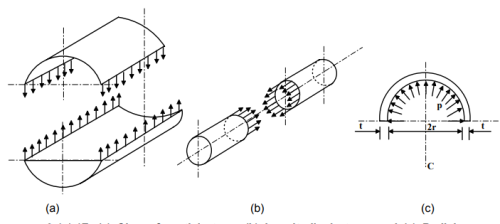9.1.1.1F- (a) Circumferential stress (b) Longitudinal stress and (c) Radial stress developed in thin cylinders.

In a thin walled cylinder the circumferential stresses may be assumed to be constant over the wall thickness and stress in the radial direction may be neglected for the analysis. Considering the equilibrium of a cut out section the circumferential stress σθ and longitudinal stress σz can be found. Consider a section of thin cylinder of radius r, wall thickness t and length L and subjected to an internal pressure p as shown in figure-9.1.1.2(a). Consider now an element of included angle dθ at an angle of θ from vertical. For equilibrium we may write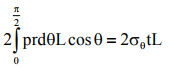This gives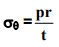Considering a section along the longitudinal axis as shown in figure-9.1.1.2 (b)

we may write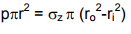where ri and ro are internal and external radii of the vessel and since ri ≈ ro = r (say) and ro – ri = t we have σz =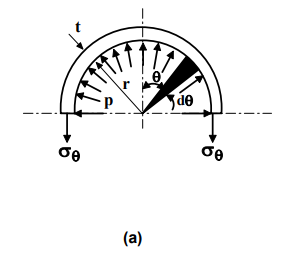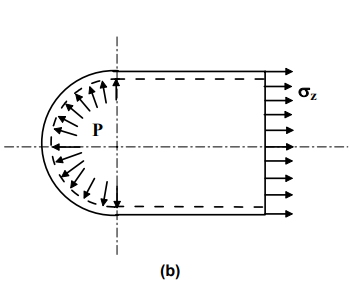9.1.1.2F- (a) Circumferential stress in a thin cylinder (b) Longitudinal stress in a thin cylinder

Thin walled spheres are also sometimes used. Consider a sphere of internal radius r subjected to an internal pressure p as shown in figure-9.1.1.3. The circumferential and longitudinal stresses developed on an element of the surface of the sphere are equal in magnitude and in the absence of any shear stress due to symmetry both the stresses are principal stresses. From the equilibrium condition in a cut section we have

σ1 = σ2=  _______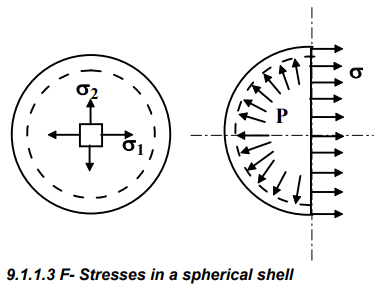Design Principles

Pressure vessels are generally manufactured from curved sheets joined by welding. Mostly V– butt welded joints are used. The riveted joints may also be used but since the plates are weakened at the joint due to the rivet holes the plate thickness should be enhanced by taking into account the joint efficiency. It is probably more instructive to follow the design procedure of a pressure vessel. We consider a mild steel vessel of 1m diameter comprising a 2.5 m long cylindrical section with hemispherical ends to sustain an internal pressure of ( say) 2MPa.

The plate thickness is given by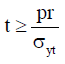where σyt is the tensile yield stress. The minimum plate thickness should conform to the “Boiler code” as given in table 9.1.2.1.

9.1.2.1T- Minimum plate thickness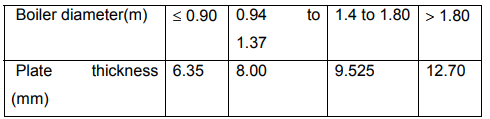The factor of safety should be at least 5 and the minimum ultimate stresses of the plates should be 385 MPa in the tension, 665 MPa in compression and 308 MPa in shear.

This gives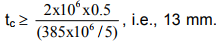Since this value is more than the value prescribed in the code the plate thickness is acceptable. However for better safety we take tc =15mm. Thickness ts of the hemispherical end is usually taken as half of this value and we take ts≈ 8mm.

Welded Joint

The circumferential stress developed in the cylinder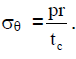With p=2MPa , r=0.5m and tc = 15 mm, σθ =67 MPa and since this is well below the allowable stress of 100 MPa ( assumed) the butt welded joint without cover plate would be adequate.

Consider now a butt joint with 10mm cover plates on both sides, as shown in figure- 9.1.2.1.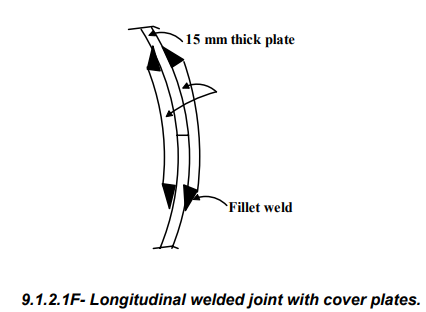The stress induced in the weld σw is given by Fc = 2σwLtcsin450 where L is the weld length. We may now write Fc = σθ t.L and therefore σw is given by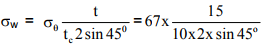which gives σw = 71 MPa which again is adequate. For increased safety we may choose the butt joint with 10mm thick cover plates. The welding arrangement of the vessel is shown in figure9.1.2.2.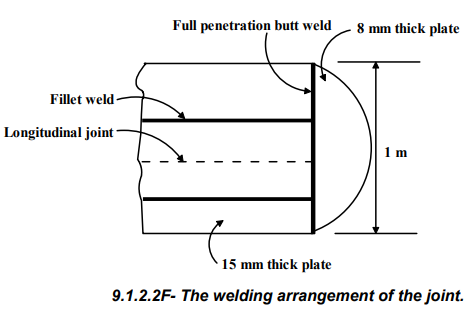Riveted Joint

The joints may also be riveted in some situations but the design must be checked for safety. The required plate thickness must take account the joint efficiency η.

This gives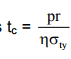Substituting p = 2MPa, r = 0.5 m, η = 70 % and σty = (385/5)

MPa we have tc = 18.5 mm. Let us use mild steel plate of 20 mm thickness for the cylinder body and 10mm thick plate for the hemispherical end cover. The cover plate thickness may be taken as 0.625tc i.e. 12.5 mm. The hoop stress is

now given by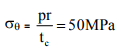and therefore the rivets must withstand σθtc i.e. 1

MN per meter.

We may begin with 20mm diameter rivets with the allowable shear and bearing stresses of 100 MPa and 300 MPa respectively. This gives bearing load on a single rivet Fb = 300x106 x0.02x0.02 = 120 kN. Assuming double shear

the shearing load on a single rivet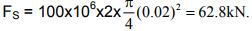The rivet pitch based on bearing load is therefore (120 kN/ 1MN per meter) i.e. 0.12m and based on shearing load is (62.8 kN/ 1MN per meter) i.e. 0.063m. We may therefore consider a minimum allowable pitch of 60mm. This gives approximately 17 rivets of 20 mm diameter per meter. If two rows are used the pitch is doubled to 120mm. For the hemispherical shaped end cover the bearing load is 60 kN and therefore the rivet pitch is again approximately 60 mm.

The maximum tensile stress developed in the plate section is σt = 1x106 /[(1-17x0.02)x0.02] = 75.76 MPa which is a safe value considering the allowable tensile stress of 385 MPa with a factor of safety of 5. A longitudinal riveted joint with cover plates is shown in figure–9.1.2.3 and the whole riveting arrangement is shown in figure-9.1.2.4.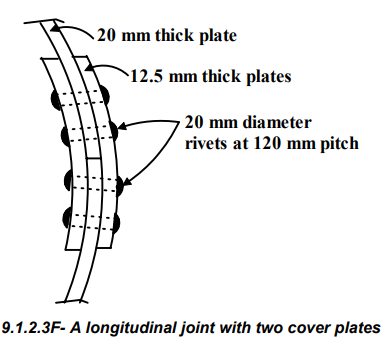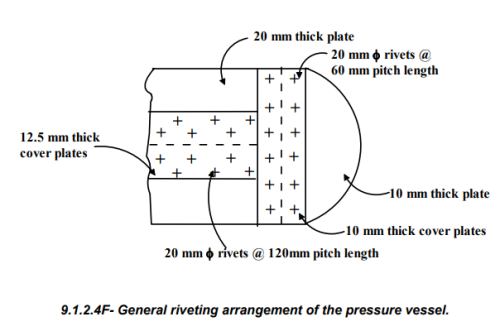Offer running on EduRev: Apply code STAYHOME200 to get INR 200 off on our premium plan EduRev Infinity!

## Strength of Materials (SOM)

28 videos|14 docs|43 tests

,

,

,

,

,

,

,

,

,

,

,

,

,

,

,

,

,

,

,

,

,

;